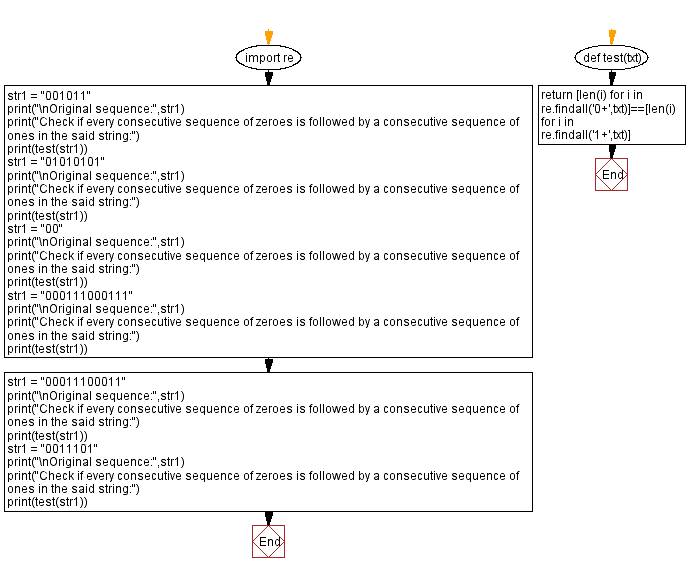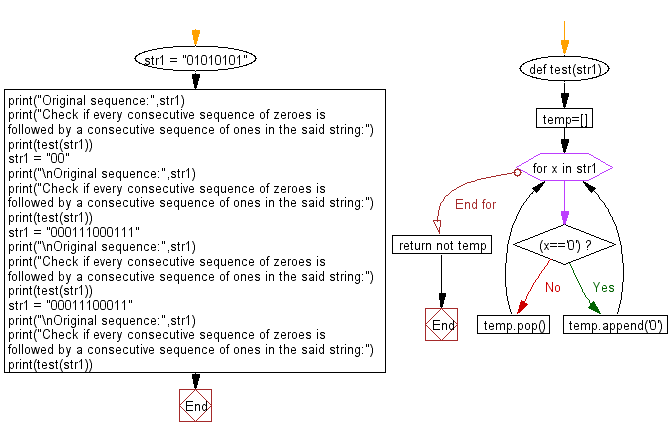﻿ Python: Check if every consecutive sequence of zeroes is followed by a consecutive sequence of ones of same length - w3resource# Python: Check if every consecutive sequence of zeroes is followed by a consecutive sequence of ones of same length

## Python Basic - 1: Exercise-142 with Solution

Write a Python program to check if every consecutive sequence of zeroes is followed by a consecutive sequence of ones of same length in a given string. Return True/False.

Sample Solution-1:

Python Code:

``````def test(str1):
while '01' in str1:
str1 = str1.replace('01', '')
return len(str1) == 0

str1 = "01010101"
print("Original sequence:",str1)
print("Check if every consecutive sequence of zeroes is followed by a consecutive sequence of ones in the said string:")
print(test(str1))
str1 = "00"
print("\nOriginal sequence:",str1)
print("Check if every consecutive sequence of zeroes is followed by a consecutive sequence of ones in the said string:")
print(test(str1))
str1 = "000111000111"
print("\nOriginal sequence:",str1)
print("Check if every consecutive sequence of zeroes is followed by a consecutive sequence of ones in the said string:")
print(test(str1))
str1 = "00011100011"
print("\nOriginal sequence:",str1)
print("Check if every consecutive sequence of zeroes is followed by a consecutive sequence of ones in the said string:")
print(test(str1))
``````

Sample Output:

```Original sequence: 01010101
Check if every consecutive sequence of zeroes is followed by a consecutive sequence of ones in the said string:
True

Original sequence: 00
Check if every consecutive sequence of zeroes is followed by a consecutive sequence of ones in the said string:
False

Original sequence: 000111000111
Check if every consecutive sequence of zeroes is followed by a consecutive sequence of ones in the said string:
True

Original sequence: 00011100011
Check if every consecutive sequence of zeroes is followed by a consecutive sequence of ones in the said string:
False
```

Flowchart:## Visualize Python code execution:

The following tool visualize what the computer is doing step-by-step as it executes the said program:

Sample Solution-2:

Python Code:

``````def test(str1):
temp=[]
for x in str1:
if (x=='0'):
temp.append('0')
else:
temp.pop()
return not temp

str1 = "01010101"
print("Original sequence:",str1)
print("Check if every consecutive sequence of zeroes is followed by a consecutive sequence of ones in the said string:")
print(test(str1))
str1 = "00"
print("\nOriginal sequence:",str1)
print("Check if every consecutive sequence of zeroes is followed by a consecutive sequence of ones in the said string:")
print(test(str1))
str1 = "000111000111"
print("\nOriginal sequence:",str1)
print("Check if every consecutive sequence of zeroes is followed by a consecutive sequence of ones in the said string:")
print(test(str1))
str1 = "00011100011"
print("\nOriginal sequence:",str1)
print("Check if every consecutive sequence of zeroes is followed by a consecutive sequence of ones in the said string:")
print(test(str1))
``````

Sample Output:

```Original sequence: 01010101
Check if every consecutive sequence of zeroes is followed by a consecutive sequence of ones in the said string:
True

Original sequence: 00
Check if every consecutive sequence of zeroes is followed by a consecutive sequence of ones in the said string:
False

Original sequence: 000111000111
Check if every consecutive sequence of zeroes is followed by a consecutive sequence of ones in the said string:
True

Original sequence: 00011100011
Check if every consecutive sequence of zeroes is followed by a consecutive sequence of ones in the said string:
False
```

Flowchart:## Visualize Python code execution:

The following tool visualize what the computer is doing step-by-step as it executes the said program:

Python Code Editor:

Have another way to solve this solution? Contribute your code (and comments) through Disqus.

What is the difficulty level of this exercise?

Test your Programming skills with w3resource's quiz.

﻿

## Python: Tips of the Day

How to make a flat list out of list of lists?

Given a list of lists l

```flat_list = [item for sublist in l for item in sublist]
```

which means:

```flat_list = []
for sublist in l:
for item in sublist:
flat_list.append(item)
```

is faster than the shortcuts posted so far. (l is the list to flatten.) Here is the corresponding function:

flatten = lambda l: [item for sublist in l for item in sublist]

As evidence, you can use the timeit module in the standard library:

```\$ python -mtimeit -s'l=[[1,2,3],[4,5,6], , [8,9]]*99' '[item for sublist in l for item in sublist]'
10000 loops, best of 3: 143 usec per loop
\$ python -mtimeit -s'l=[[1,2,3],[4,5,6], , [8,9]]*99' 'sum(l, [])'
1000 loops, best of 3: 969 usec per loop
\$ python -mtimeit -s'l=[[1,2,3],[4,5,6], , [8,9]]*99' 'reduce(lambda x,y: x+y,l)'
1000 loops, best of 3: 1.1 msec per loop
```

Explanation: the shortcuts based on + (including the implied use in sum) are, of necessity, O(L**2) when there are L sublists -- as the intermediate result list keeps getting longer, at each step a new intermediate result list object gets allocated, and all the items in the previous intermediate result must be copied over (as well as a few new ones added at the end). So, for simplicity and without actual loss of generality, say you have L sublists of I items each: the first I items are copied back and forth L-1 times, the second I items L-2 times, and so on; total number of copies is I times the sum of x for x from 1 to L excluded, i.e., I * (L**2)/2.

The list comprehension just generates one list, once, and copies each item over (from its original place of residence to the result list) also exactly once.

Ref: https://bit.ly/3dKsNTR